# How to get the FY19 and the FY20 values in the same Line Item.edited February 27 in Modeling

Hi need help on the below query.

I have three line items.

A, B, C where in A and B are the inputs and the C is based on the Formula.

IF (PREVIOUS(C) + A +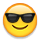< 0 THEN -(PREVIOUS(C) + A +* 0.0386 ELSE (PREVIOUS(C) + A +* 0.0386

The Function previous gives the value of the previous month. But I want the value of FY19 in the place of PREVIOUS(C) value. The FY19 value which I want in place of PREVIOUS(C) is fixed for the all the months of FY20 and the value of Dec20 is fixed for all the months of the FY21.

Note : The values of FY19 and FY20 are not the sum of the full year.

I'm not getting the idea about how to get the value of FY19 in the place of previous(c) - in the above formula.

I have attached the excel screenshot and Anaplan screenshot.

Looking forward for the help.

Tagged:

•Would YearValue() not work for you?

IF PREVIOUS(YEARVALUE(C)) + A + B < 0 THEN -(PREVIOUS(YEARVALUE(C)) + A +* 0.0386 ELSE (PREVIOUS(YEARVALUE(C)) + A +* 0.0386

Rob

•This will not work as I will get the circular reference error. The screenshot that I have attached where in I'm adding this formula in the Line Item C.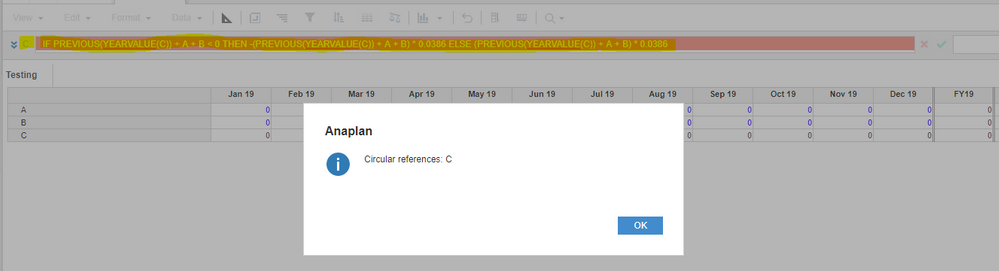•@riyazpasha If I understand your issue then you are trying to pull the December number into the next year, aka the closing balance is the summary method?

I have solved this by doing the following: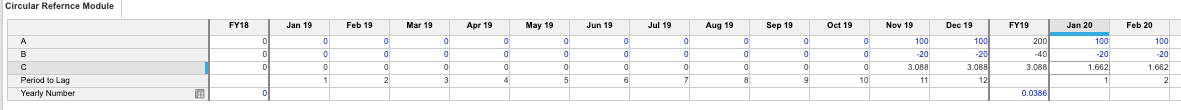I have added a Line item Called "Period to Lag" Which is just calling out the month of the year using MONTH(ITEM(TIME)) as the formula (This would probably be better suited in a time settings System module than here)

I added another line item Called "Yearly Number" that allows the .0386 or the .02 to be inputs by year since it appears they change by year.

A and B are still inputs per your request, and C I have simplified:

In your original formula, it seems like you want it to be a positive number even if it calculates as a negative, so instead of using the IF statement, I have just used the ABS() formula to show the Absolute Value.

Secondly, I am using the LAG() Formula to Move the December number a certain number of periods. We can use the "Strict" Mode here to prevent the formula from having a possibility of circularity.

Here is what my final formula looks like: ABS(A + B + LAG(C, Period to Lag, 0, STRICT)) * YEARVALUE(Yearly Number)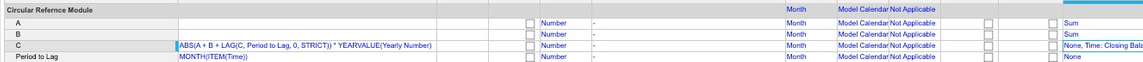My Yearly Number line item simply looks like this and is an Input: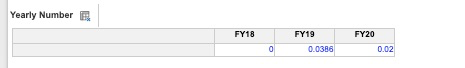Hope this helps!
Jason

•Thank you so much it worked for me. Thanks a lot.